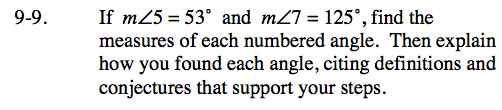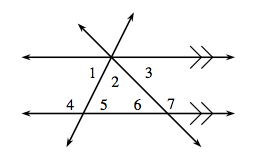9-9.

Missing problem for Book CC3 => Chapter Ch9 => Lesson 9.1.1 => Problem 9-9

Created from orphaned homework help problemSince two of the lines are parallel, their alternate interior angles and corresponding angles are equal to each other.

Angles 1, 2, and 3 form a straight angle, as do angles 4 and 5 and angles 6 and 7.

m∠7 = m∠1 + m∠2